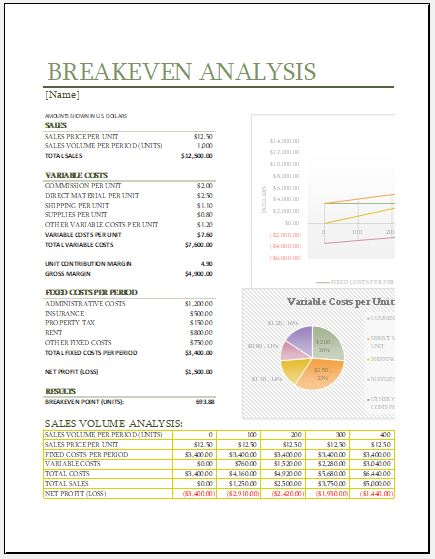# Breakeven Calculator Template

## What is a break-even calculator?

It is very important for every organization to conduct the breakeven analysis in order to check how feasible it is to launch a particular product. The tool which is used to conduct this type of analysis is known as a breakeven calculator.

There are some very basic and simple formulas which are commonly used to conduct the breakeven analysis. However, sometimes the calculation becomes a little complicated when you have to keep track of complex projected sales and other such details.

Breakeven calculator can be used by the person for his recently started business in order to check whether a particular product would be financially feasible to produce or not. It analyzes both the production and selling of the new product.

This is a very common calculator which is used in almost every solid business plan. The formulas used in this calculator are very simple, however; the difficulty in calculations can be seen in projected sales, selecting the suitable sales price and calculating the fixed and variable costs.

These tasks are operated by the owner of the business. The break-even calculator helps the person running his business smoothly.

The break-even calculator is a very simple spreadsheet template which has three worksheets in it to solve break-even units, breakeven price, and payback period. With all these three worksheets, you can evaluate the simple break-even equation.

The breakeven points are solved by this spreadsheet quickly. Moreover, the number of units is also solved by this calculator.

The formulas which have been used in this calculator are based on the model of linear cost volume. The spreadsheet template also uses the breakeven charts which shows these points as the point of intersection of the total revenue and total cost when they are plotted on the graph.

You can easily find the formula in it, however; the most commonly used formula is the one which calculates the breakeven units. The breakeven units may also mean the number of units which you may have to sell in order to cover the cost.

## What is the main purpose of using the break-even calculator?

The main purpose of using this calculator for most of the companies is to get information about how they can cover the cost of manufacturing the product. Usually, companies are required to sell a specific number of products in order to cover the cost. How many units they should sell for covering the cost can be determined easily with the help of a breakeven calculator.

In simple words, the payback period can be evaluated by using Break even calculator. The breakeven calculator template is the best way to amount of time needed to break even. There are many simple as well as complex formulas pre-defined in the template that save the time and efforts of the user.

## The template

The template is prepared in Microsoft Excel format in the form of a readymade spreadsheet with default formulas for quick and accurate calculations. The user is required to input the cost he has incurred only. The cost the user should input includes both variable and fixed costs. The template quickly calculates the total number of units a company should sell for getting the cost back. This template is highly useful for small retail stores and for online stores.

It is very important for every retail business to discover when he will be able to cover the costs and start to make a profit. The breakeven points can be determined by making use of a breakeven calculator. Moreover, the calculation results can also be used in order to make financial decisions.

Preview### Breakeven CalculatorTemplate

Format: Microsoft Excel 2007-2013

File Size: 33 KB